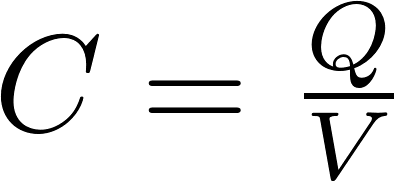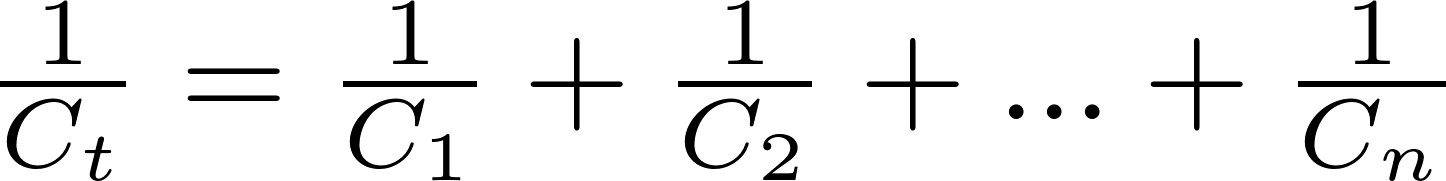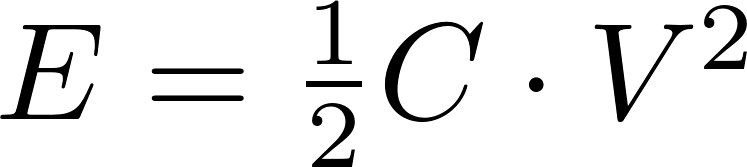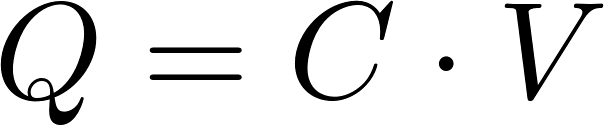# Capacitor

The capacitor is a passive electrical component that stores energy in an electric field. This effect is called capacitance. The unit for capacitance is the farad (F), which is defined as one coulomb per volt (1 C/V). Most capacitors consist of two metal plates separated by a dielectric medium. When a voltage is applied, one of the plates will get a negative charge and the other one a positive charge.

### Capacitance

Capacitance calculator

The capacitance (C) is equal to the charge (Q) divided by the voltage (V). You can calculate the capacitance with the following formula:C is the symbol for capacitance and is measured in farad (F).
Q is the symbol for charge and is measured in coulomb (C).
V is the symbol for voltage and is measured in volt (V).

### Capacitors in series

Capacitors in series

Capacitors can be connected in series (one after another). The capacitance is equal to the reciprocal (inverse) of the sum of the reciprocal of all individual capacitances.### Capacitors in parallel

Capacitors in parallel

Capacitors can also be connected in parallel. The capacitance in this situation is equal to the sum of all individual capacitances.### Markings

Capacitor codes

Most small capacitors use three digits (and sometimes a letter) to indicate the capacitance. the capacitance in picofarad (pF) is equal to the first two digits multiplied by ten to the power of the third digit. The letter at the end indicates the tolerance.

letter tolerance letter tolerance
A 0.05pF H 3%
B 0.1pF J 5%
C 0.25pF K 10%
D 0.5pF L 15%
E 0.5% M 20%
F 1% V 25%
G 2% N 30%
Z +80% -20% - -

### Energy and charge stored in a capacitor

Energy and charge stored in a capacitor

Both the stored energy and stored charge can be calculated if the capacitance and voltage are known. They can be calculated with the following formulas:E is the symbol for energy and is measured in joule (J).
C is the symbol for capacitance and is measured in farad (F).
V is the symbol for voltage and is measured in volt (V).
Q is the symbol for charge and is measured in coulomb (C).